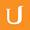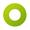• 0:00 - 0:04
In homework 3.4, you're asked to simulate circular robotic motion.
• 0:04 - 0:08
• 0:08 - 0:12
I want to give you those formulas again and explain where some of them came from.
• 0:12 - 0:17
The first equation I want to talk about is this one.
• 0:17 - 0:23
The radius of curvature is equal to the length of the vehicle over the tangent of alpha
• 0:23 - 0:27
where alpha is our steering angle. Let me write that up here.
• 0:27 - 0:30
So where does this equation come from.
• 0:30 - 0:36
To derive it, the key realization is that the front and rear tire do not travel along the same circle.
• 0:36 - 0:40
Here's my rear tire, and here's my front tire.
• 0:40 - 0:45
They are, of course, separated by a distance that we called "L."
• 0:45 - 0:49
Let's draw the circles that these tires travel along.
• 0:49 - 0:56
Well, this rear tire is actually going to travel along a smaller inner circle
• 0:56 - 0:59
while this tire is going to travel along a larger outer circle.
• 0:59 - 1:04
Since we defined our radius of curvature as the distance from the back tire to the center,
• 1:04 - 1:11
Let's label this r, and we can see that the line connecting these tires defines an axis,
• 1:11 - 1:16
and here we have our steering angle, alpha, from here.
• 1:16 - 1:18
Now we can do a little bit of geometry.
• 1:18 - 1:20
Let's make a right triangle.
• 1:20 - 1:26
Well, if this angle here is alpha, then this much be a 90 degree angle,
• 1:26 - 1:30
because a radius intersecting with a tangent line always forms a right angle.
• 1:30 - 1:37
That means that that this angle here must be equal to 90 degrees minus alpha,
• 1:37 - 1:43
which means this angle, since this is a right triangle must be alpha.
• 1:43 - 1:47
Well, we're almost there. The tangent of this angle is equal to the opposite side,
• 1:47 - 1:51
which is the length, over the adjacent side, which is the radius.
• 1:51 - 1:56
So tangent of alpha is equal to L over r.
• 1:56 - 2:00
We manipulate this equation a little bit, and we find that the radius of curvature
• 2:00 -
is equal to the length of the vehicle over the tangent of the steering angle.
タイトル：

dummy description

more » « less
Team:Udacity
プロジェクト：
CS373 - Artificial Intelligence
Duration:
02:08Amara Bot added a translation

• Amara Bot